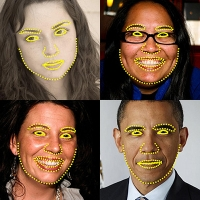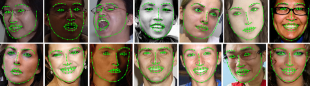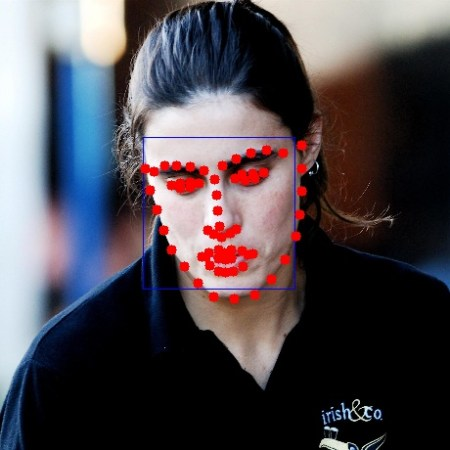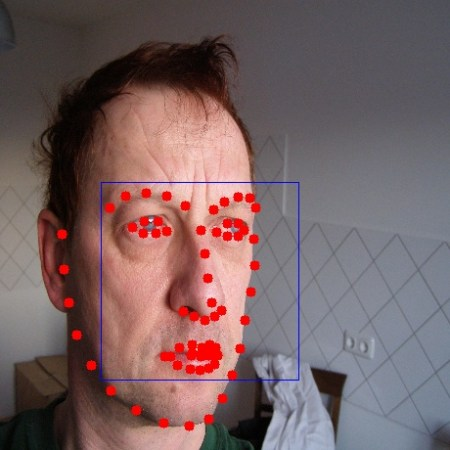OpenCV  4.0.0 Open Source Computer Vision
Face landmark detection in an imageThis application lets you detect landmarks of detected faces in an image. You can detect landmarks of all the faces found in an image and use them further in various applications like face swapping, face averaging etc. This functionality is now available in OpenCV.

// Command to be typed for running the sample

### Description of command parameters {tutorial_face_training_parameters}

• model_filename f : (REQUIRED) A path to binary file storing the trained model which is to be loaded [example - /data/file.dat]
• image i : (REQUIRED) A path to image in which face landmarks have to be detected.[example - /data/image.jpg]
• face_cascade c : (REQUIRED) A path to the face cascade xml file which you want to use as a face detector.

## Understanding codeThis tutorial will explain the sample code for face landmark detection. Jumping directly to the code :

Ptr<Facemark> facemark = createFacemarkKazemi());

The above code creates a CascadeClassifier to detect face regions, and an instance of the face landmark detection class. We need to load a pretrained model for face landmark detection, and a cascade file for the face detection. It also loads the image in which landmarks have to be detected.

vector<Rect> faces;
resize(img,img,Size(460,460),0,0,INTER_LINEAR_EXACT);
Mat gray;
std::vector<Rect> faces;
if(img.channels()>1){
cvtColor(img.getMat(),gray,COLOR_BGR2GRAY);
}
else{
gray = img.getMat().clone();
}
equalizeHist( gray, gray );
face_cascade.detectMultiScale( gray, faces, 1.1, 3,0, Size(30, 30) );

After doing some preprocessing, we first have to detect possible face regions (which will be stored in a vector<Rect>. Also, the image is resized to a smaller size as processing speed is faster with small images.

vector< vector<Point2f> > shapes;
if (facemark->fit(img,faces,shapes))
{
for ( size_t i = 0; i < faces.size(); i++ )
{
cv::rectangle(img,faces[i],Scalar( 255, 0, 0 ));
}
for (unsigned long i=0;i<faces.size();i++){
for(unsigned long k=0;k<shapes[i].size();k++)
cv::circle(img,shapes[i][k],5,cv::Scalar(0,0,255),FILLED);
}
namedWindow("Detected_shape");
imshow("Detected_shape",img);
waitKey(0);
}

It then creates a vector of vector to store shapes for each face detected. The above code calls the function fit to get shapes of all detected faces in the image and then draws the rectangles bounding the faces and marks the desired landmarks.

### Detection Results